# Olympiad Test Level 2: Patterns

## 15 Questions MCQ Test Mathematics Olympiad for Class 3 | Olympiad Test Level 2: Patterns

Description
Attempt Olympiad Test Level 2: Patterns | 15 questions in 30 minutes | Mock test for Class 3 preparation | Free important questions MCQ to study Mathematics Olympiad for Class 3 for Class 3 Exam | Download free PDF with solutions
QUESTION: 1

### Find out the missing term in the given alphabetical pattern?AA, BB, CC, ? , EE, FF

Solution:

In the series, the terms AA, BB and CC are repeated in alphabetical order.

QUESTION: 2

### Find the missing number in the number sequence given below?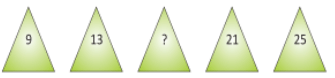Solution: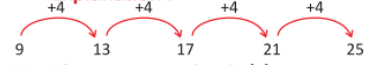QUESTION: 3

### Find out the missing number in the given number pattern?11, 22, ? , 44, 55, 66

Solution:

Multiples of 11 i.e., 11, 22, 33, 44, 55, 66.

QUESTION: 4

Find the next alphabet in the given alphabetic pattern?

A, D, G, J, M, ?

Solution: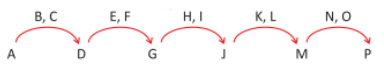QUESTION: 5

Find the missing numbers that will complete the given chart.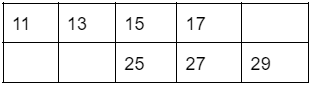Solution:

In the table, 2 is added in the previous number to get the next number.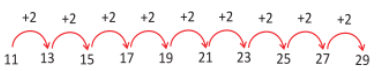QUESTION: 6

Which numbers will come next? 4,9,14,19,24, ?, 34,39,44, ? ,54,59, ?, 69,74,79,84, ?

Solution:

Add 5 to the previous number to get the next number in the pattern. Check the encircled numbers.

4 + 5 = 9, 9 + 5 = 14, 14 + 5 = 19, 19 + 5 = 24, 24 + 5 = 29 ,

29 + 5 34,34+5=39, 39+5=44,44+5=49, 49 + 5 = 54, 54 + 5 = 59, 59 + 5 = 64 ,

64 + 5 = 69, 69 + 5 = 74, 74 + 5 = 79, 79 + 5 = 84, 84 + 5 = 89.

QUESTION: 7

Find the next figure in the given figure pattern.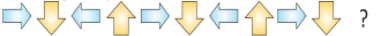Solution:

The pattern in following as there is the right arrow first, then the down arrow, then the left arrow and then the upward arrow following the same sequence.

So, the missing arrow next to the down arrow is the left arrow.

QUESTION: 8

Anjana made the number pattern below.

4, 8, 12, , , ,28

What three numbers are missing from the pattern?

Solution:

In the pattern, add 4 to the previous number to get the next number.

Or

4, 4 + 4 = 8, 8 + 4 = 12, 12 + 4 = 16. 16 + 4 = 20, 20 + 4 = 24, 24 + 4 = 28.

QUESTION: 9

Mrs. Kavita is lining up her students in this order:

girl, boy, boy, girl, boy, boy, girl, boy, boy, girl, boy, , .

If she continues the same pattern, what are the next two students in line?

Solution:

Consider the 1st three student’s line up....boy, boy follow the girl.

girl, boy, boy | girl, boy, boy | girl, boy, boy | girl, boy, boy|girl, boy, boy |

QUESTION: 10

Find the next figure in the given pattern?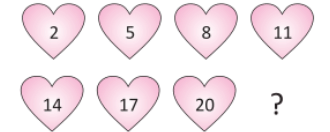Solution:

The pattern is as follows: Add 3 to the previous number in the heart shape to get the next number.

2, 2 + 3 = 5, 5 + 3 = 8, 8 + 3 = 11,

11 + 3 = 14, 14 + 3 = 17, 17 + 3 = 20,

20 + 3 = 23.

QUESTION: 11

Complete the given number pattern.Solution:

The pattern is... add 3 to the previous number in the shape to get the next number.

3, 3 + 3 = 6, 6 + 3 = 9, 9 + 3 = 12,

12 + 3 = 15, 15 + 3 = 18.

QUESTION: 12

How many hearts will be there in pattern 6?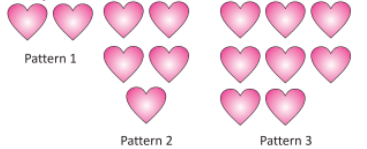Solution:

In every term there are 3 hearts more than the previous term.

Therefore the 4th term will have 11 (8+3) hearts, the 5th term will have 14 (11+3) hearts and the 6th pattern will have 17 (14+3)hearts.

QUESTION: 13

Find the missing number in the given number pattern?

14, 17, 20, ? , 26, 29

Solution:

3 is added to the previous number to get the next number.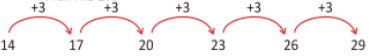QUESTION: 14

Find the next term in the given alphabetic sequence?

Aa, Bb, Cc, Dd, Ee, _?_

Solution:

Every capital letter is followed by small letter in alphabetical order

QUESTION: 15

Find the next term in the alphabetic sequence given below?

Aa, Bbb, Cccc, Ddddd, ?

Solution:

The pattern is as follows: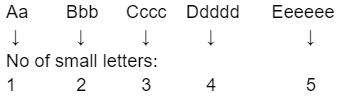Use Code STAYHOME200 and get INR 200 additional OFF Use Coupon Code